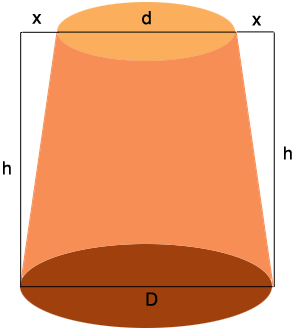SEARCH HOMEMath Central Quandaries & QueriesQuestion from Peter: I am trying to calculate the diameter of a truncated cone given one diameter the height of the cone and a 10% taper from one end to the other. For example a butter churn is 18" tall and 9" in diameter at the base. the sides need to slope inward at 10% What is the diameter at 9" and 18"Hi Peter,

I drew a diagram with the diameter of the base $D$ inches, the diameter of the top $d$ inches and the height $h$ inches.Since the taper is 10% the quantity $x$ in the diagram is 105 of $h,$ that is $x = 0.1 \times h.$ Thus

$d = D - 2h = D - 0.2 \times h.$

PennyMath Central is supported by the University of Regina and the Imperial Oil Foundation.